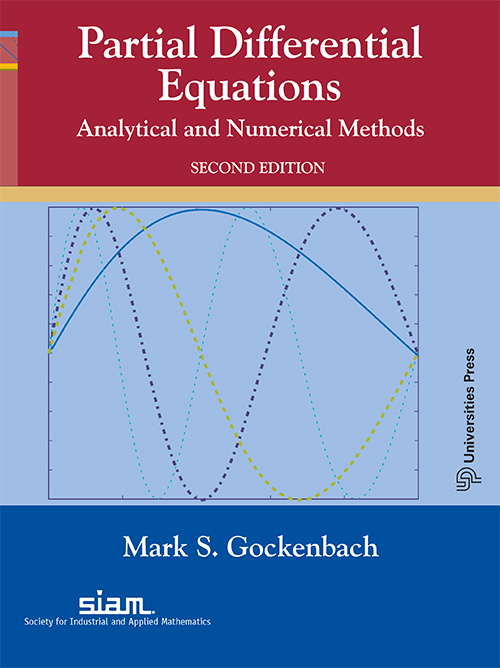## Partial Differential Equations: Analytical and Numerical Methods, Second Edition

#### Price: 1275.00

This undergraduate textbook introduces students to the topic with a unique approach that emphasizes the modern finite element method alongside the classical method of Fourier analysis.

Additional features of this new edition include

• broader coverage of PDE methods and applications, with new chapters on the method of characteristics, Sturm–Liouville problems, and Green's functions, a new section on the finite difference method for the wave equation;
• emphasis on linear algebra, particularly the idea of best approximation;
• realistic physical parameters and meaningful experiments for many of the examples and exercises;
• tutorials for the most popular software (MATLAB™, Mathematica™, and Maple™) that can be used to reproduce the examples and solve the exercises.

The author continues to emphasize  Fourier series and finite element methods, which were the primary scope of the first edition.

This book is written for undergraduate courses usually titled Introduction to Partial Differential Equations or Fourier Series and Boundary Value Problems.

Keywords: partial differential equations (PDEs), finite element method, Fourier analysis, finite difference method, linear algebra, MATLAB™, Mathematica™, and Maple™

#### Contributors (Author(s), Editor(s), Translator(s), Illustrator(s) etc.)

Mark S. Gockenbach is Professor and Chair of the Department of Mathematical Sciences at Michigan Technological University. He is the author of Partial Differential Equations: Analytical and Numerical Methods (SIAM, 2002), Understanding and Implementing the Finite Element Method (SIAM, 2006), and Finite-Dimensional Linear Algebra (CRC Press, 2010). His research interests include inverse problems in PDEs and numerical methods and software for large-scale optimization problems.